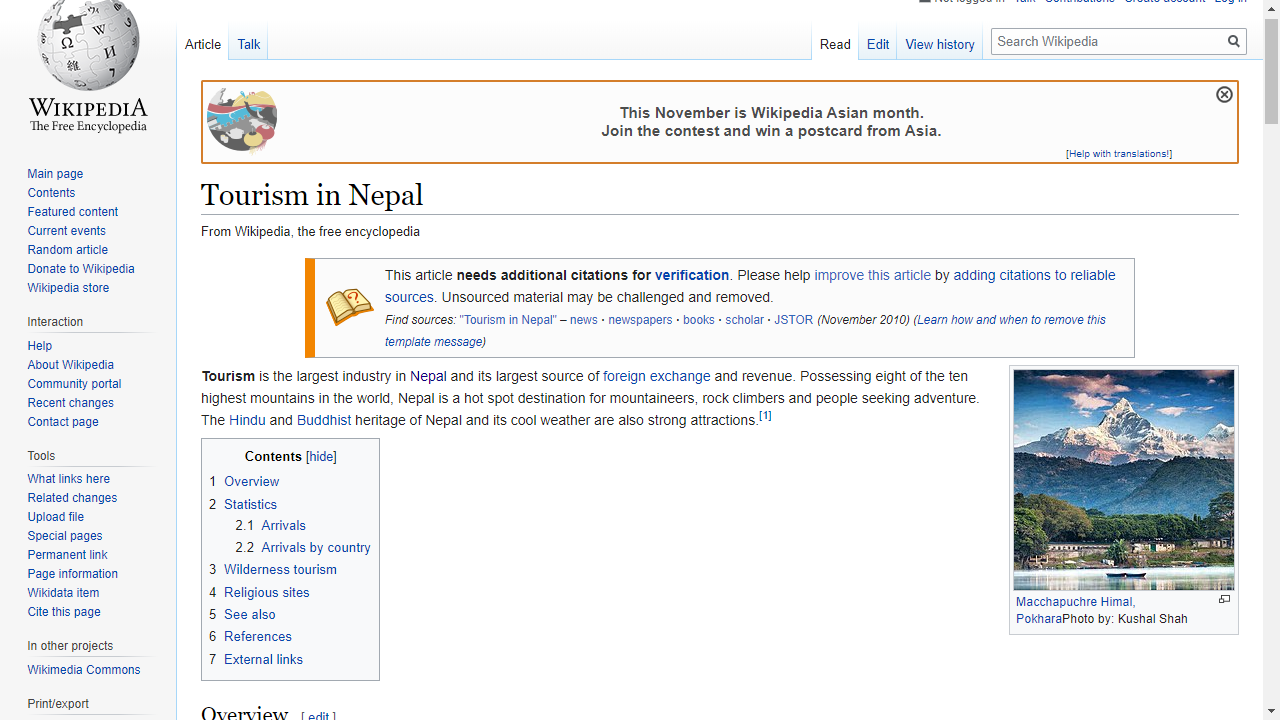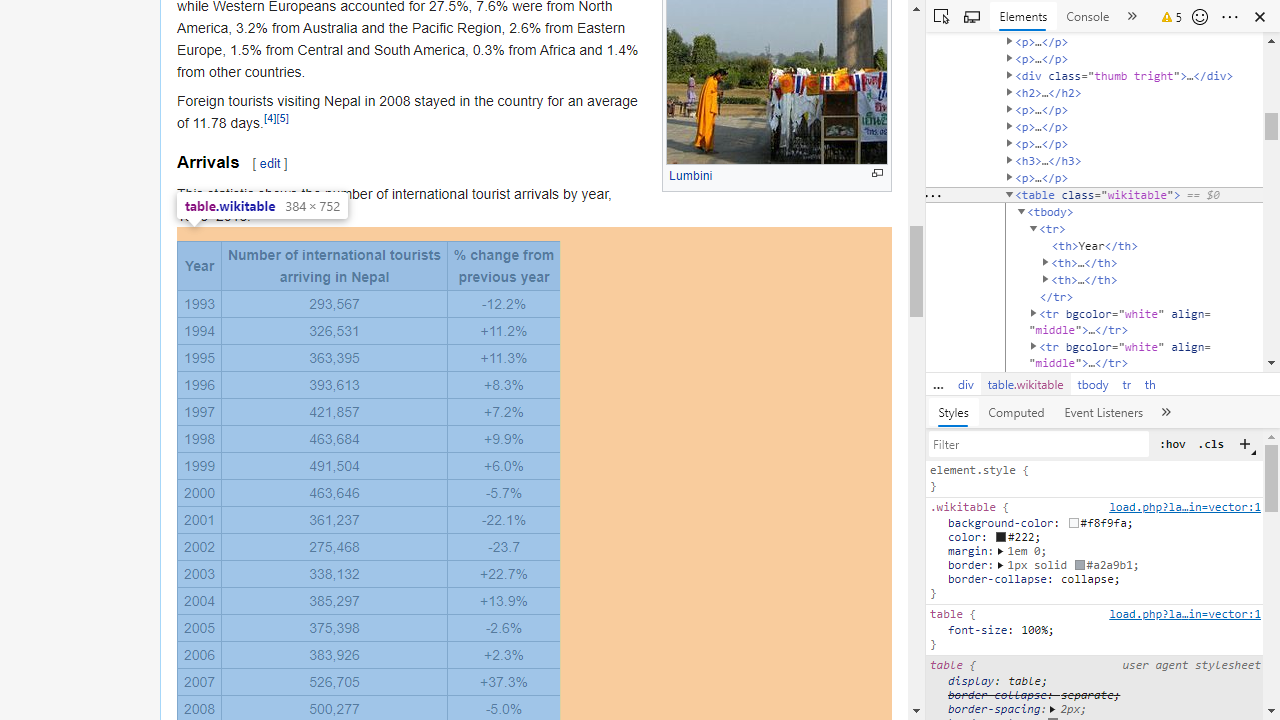# Scraping Data From Wikipedia With R

In Data Analysis require a data on which we can perform analysis and find insights. We always don’t get clean open data for our analysis so we need to create, find our data. In this post, I describe how to extract data from a website using R.

Diwash Shrestha https://diwashrestha.com.np
11-05-2019Nepal is a Himalayan nation with beautiful mountains, ancient cultures, traditions and rich history. Nepal is celebrating year 2020 as “Tourism Year” targeting 2 million international tourist arrivals. You can learn more about the #VisitNepal2020.

I wanted to find out the history of international tourist arrival in Nepal and found data in Wikipedia I wanted to extract the data from Wikipedia and analyse and create some visualization and reports. This post will highlight how I got to scraping out this data using R’s package rvest. rvest is an R package that makes it easy for us to scrape data from the web.

## Lets StartAbove figure shows the website from which I will extract the data.

I will import the rvest package which makes it easy to scrape (or harvest) data from HTML web pages.

``````
library(rvest)
library(stringr)
library(tidyverse)``````

We need the link from which we want to scrape the data. I opened the wiki webpage and I will scrape the table shown below.

I will use the read_html() function which reads the HTML page.

``````
wikipage <- read_html("https://en.wikipedia.org/wiki/Tourism_in_Nepal")``````

## Data Extraction

We need the CSS selector of the table from which we want to scrape the data. I opened the inspect view which can be opened by clicking the right button of the webpage and click the inspect. for the table, the CSS selector is “table.wikitable”.I passed the CSS selector to the html_nodes() function and result is passed to the html_table() function using pipeline(%>%). The below code extract the arrival table data as data frame.

``````
table <- wikipage %>%
html_nodes("table.wikitable") %>%
html_table(header=T)
table <- table[]

#add the table to a dataframe
tourist_df <- as.data.frame(table)``````

I will change the column to a short form so that it will be easy to work on it.

``````
names(tourist_df) <- c("year","tourist_number","per_change")``````

The tourist_number column has “,” between the number which makes it string. I removed the “,” using str_remove() from stringr package.

``````
tourist_df\$tourist_number <- str_remove(tourist_df\$tourist_number,",")
tourist_df\$per_change <- str_remove(tourist_df\$per_change, "%")
tourist_df\$tourist_number <- str_remove(tourist_df\$tourist_number,",")``````

As the extracted data was string, I convert columns to integer and numeric type.

``````
tourist_df\$tourist_number <- as.integer(tourist_df\$tourist_number)
tourist_df\$per_change <- as.numeric(tourist_df\$per_change)
tourist_df\$year <- as.integer(tourist_df\$year)``````

This is the final dataframe after cleaning.

In the same way, I can extract another arrival from the country table. both tables have the same selector “table.wikitable” when the html_nodes extract the tables it keeps data in list form in the table object. We can extract the data for the arrival by country from table list using the index 2.

``````
table <- wikipage %>%
html_nodes("table.wikitable") %>%
html_table(header=T)
table <- table[]

#add the table to a dataframe
con_tour_df <- as.data.frame(table)``````

I rename the columns of the dataframe .

``````
names(con_tour_df)<- c("Rank","Country",2013,2014,2015,2016,2017)``````

This the data how it look like after extracting using rvest.

I remove the “,” from every column using the purr package map() and str_remove() of the stringr package.

``````
con_tour_df <- con_tour_df%>% map(str_remove,",") %>% as_tibble()``````

This is the final dataframe after some cleaning process.

Finally, I have extracted the data from Wikipedia. I will analyse this data and share the insights in the next post.

Feel free to send to me your feedback and suggestions regarding this post!

An introduction to web scraping using R

Analytics Vidhya, Beginner’s guide on web scraping